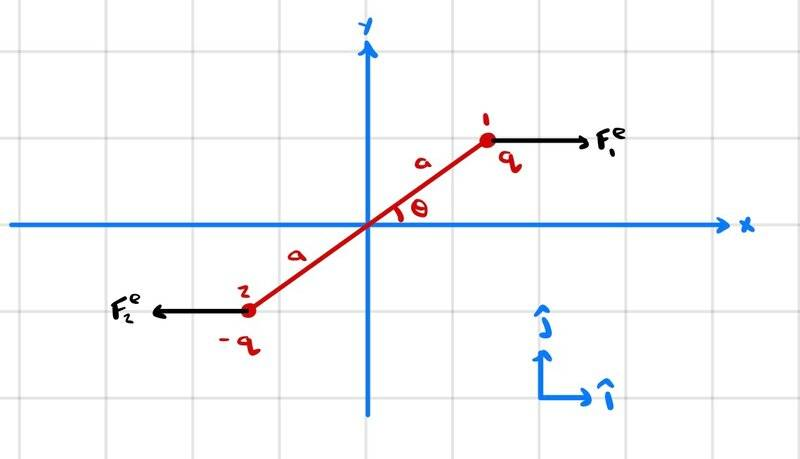# Solving for Simple Harmonic Motion: A Picture Problem

• zenterix

#### zenterix

Homework Statement
An electric dipole lying in the xy-plane with a uniform electric field applied in the positive x-direction is displaced by a small angle ##\theta## from its equilibrium position. The charges are separated by a distance ##2a##. The moment of inertia of the dipole about the center of mass is ##I_{cm}##.

If the dipole is released from this position, show that its angular orientation exhibits simple harmonic motion. What is the period of oscillation?
Relevant Equations
$$\vec{\tau}=-2aE_x\sin{\theta} \hat{k}$$

$$\tau_z=I_{cm}\alpha_z$$

$$\alpha_z=\frac{\tau_z}{I_{cm}}=\frac{-2aE_x\sin{\theta}}{I_{cm}}=-B\sin{\theta}$$

where $$B=\frac{2aE_x}{I_{cm}}$$

This is a differential equation. I think that solving this equation would provide the correct result, but I don't want to go this route.

One route is, if ##\theta## is small, to use the approximation ##\sin{\theta}\approx\theta##.

Then

$$\alpha_z(t)=\theta''(t)=-B\theta(t)$$

I think this is the differential equation representing a simple harmonic motion of an ideal spring with

$$B=\frac{k}{m}$$
Here is a picture of the problemIt is not clear to me how to really prove that the equation for ##\theta(t)## is simple harmonic motion, and what the period of this motion is.

Your equation is the equation for simple harmonic motion which is of the form ##~\ddot y=-\omega^2 y~## where ##\omega## is a real constant and the differentiation is with respect to time. Any equation in that form describes simple harmonic motion. Do you know what the most general solution of that is? If so, plug it in and you should be able to deduce the period of the motion.